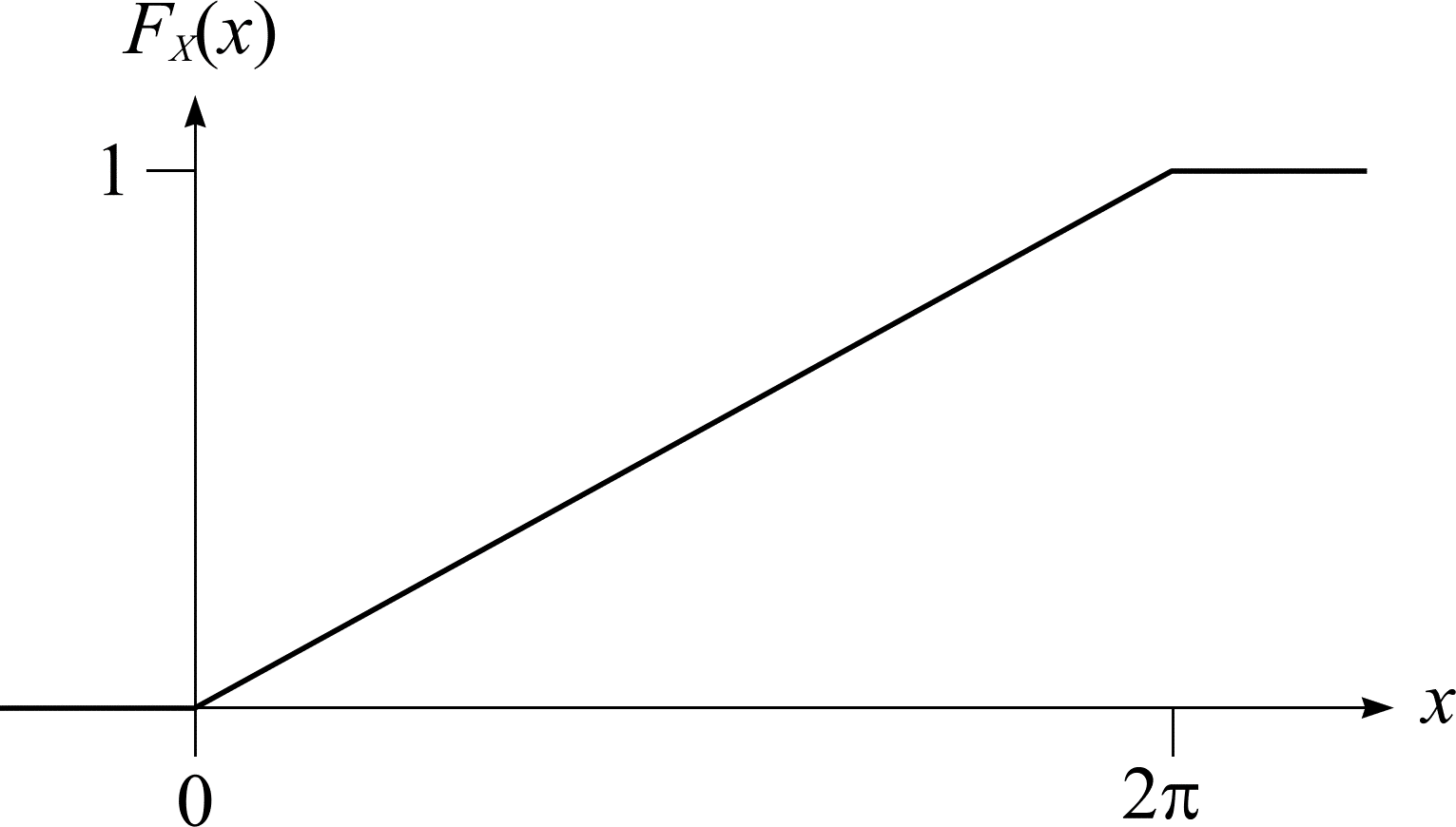# Continuous random variables

The random variable $$X$$ is continuous if its distribution function can be expressed as

\begin{align} F_{X}(x) &= \mathbf{P}(X \le x) \\ &= \int_{-\infty}^{x}f_{X}(u)\mathrm{d}u, & x \in \real, \end{align} \tag{6}

for some integrable function $$f_{X} : \real \to [0, \infty)$$. In this case, $$f_{X}$$ is called the (probability) density function of $$X$$. The fundamental theorem of calculus and (6) imply

\begin{align*} \mathbf{P}(a \le X \le b) &= F_{X}(b) - F_{X}(a) \\ &= \int_{a}^{b}f_{X}(x)\mathrm{d}x. \end{align*}

$$f(x)\delta x$$ can be thought of as the element of probability $$\mathbf{P}(x \le X \le x + \delta x)$$ where

\begin{align} \mathbf{P}(x \le X \le x + \delta x) &= F_{X}(x + \delta x) - F_{X}(x) \\ &\approx f_{X}(x)\delta x. \end{align} \tag{7}
$$\mathbf{P}(x \le X \le x + \delta x) = F_{X}(x + \delta x) - F_{X}(x) \approx f_{X}(x)\delta x.$$
$$(7)$$

If $$B_{1}$$ is a measurable subset of $$\real$$ (such as a line segment or union of line segments) then

$$\mathbf{P}(X \in B_{1}) = \int_{B_{1}} f_{X}(x)\mathrm{d}x \tag{8}$$

where $$\mathbf{P}(X \in B_{1})$$ is the probability that the outcome of this random choice lies in $$B_{1}$$. The expected value (or expectation) of $$X$$ with density function $$f_{X}$$ is

$$\mu = \mathbf{E}X = \int_{-\infty}^{\infty} x f_{X}(x) \mathrm{d}x \tag{9}$$

whenever this integral exists. The variance of $$X$$ or $$\mathrm{Var}(X)$$ is defined by the already familiar (3). Since $$X$$ is continuous, (3) can be re-expressed accordingly:

\begin{align} \sigma^{2} &= \mathrm{Var}(X) \\ &= \mathbf{E}\left((X - \mu)^{2}\right) \\ &= \int_{-\infty}^{\infty}(x - \mu)^{2}f_{X}(x)\mathrm{d}x. \end{align} \tag{10}

The joint distribution function of the continuous random variables $$X$$ and $$Y$$ is the function $$F_{X, Y} : \real^{2} \to [0, 1]$$ given by $$F_{X, Y}(x, y) = \mathbf{P}(X \le x, Y \le y)$$. $$X$$ and $$Y$$ are (jointly) continuous with joint (probability) density function $$f_{X, Y} : \real^{2} \to [0, \infty)$$ if

$$F_{X, Y}(x, y) = \int_{-\infty}^{y} \int_{-\infty}^{x} f_{X, Y}(u, v)\mathrm{d}u\mathrm{d}v$$

for each $$x, y \in \real$$.

The fundamental theorem of calculus suggests the following result

\begin{align*} \mathbf{P} \left(\begin{align*} a \le X \le b,\\ c \le Y \le d \end{align*}\right) &= F_{X, Y}(b, d) - F_{X, Y}(a, d) - F_{X, Y}(b, c) + F_{X, Y}(a, c) \\ &= \int_{c}^{d}\int_{a}^{b}f_{X, Y}(x, y)\mathrm{d}x\mathrm{d}y. \end{align*}
$$\mathbf{P}(a \le X \le b, c \le Y \le d) = F_{X, Y}(b, d) - F_{X, Y}(a, d) - F_{X, Y}(b, c) + F_{X, Y}(a, c) = \int_{c}^{d}\int_{a}^{b}f_{X, Y}(x, y)\mathrm{d}x\mathrm{d}y.$$

$$f_{X, Y}(x, y)\delta x \delta y$$ can be thought of as the element of probability $$\mathbf{P}(x \le X \le x + \delta x, y \le Y \le y + \delta y)$$ where

\begin{align} \mathbf{P} \left(\begin{align*} x \le X \le x + \delta x,\\ y \le Y \le y + \delta y \end{align*}\right) &= \begin{align*} & F_{X, Y}(x + \delta x, y + \delta y) - F_{X, Y}(x, y + \delta y) \\ & - F_{X, Y}(x + \delta x, y) + F_{X, Y}(x, y) \end{align*} \\ &= f_{X, Y}(x, y) \delta x \delta y. \end{align} \tag{11}
$$\mathbf{P} (x \le X \le x + \delta x, y \le Y \le y + \delta y) = F_{X, Y}(x + \delta x, y + \delta y) - F_{X, Y}(x, y + \delta y) - F_{X, Y}(x + \delta x, y) + F_{X, Y}(x, y) = f_{X, Y}(x, y) \delta x \delta y.$$
$$(11)$$

If $$B_{2}$$ is a measurable subset of $$\real^{2}$$ (such as a rectangle or union of rectangles and so on) then

$$\mathbf{P}\left((X, Y) \in B_{2}\right) = \int \int_{B_{2}} f_{X, Y}(x, y) \mathrm{d}x\mathrm{d}y \tag{12}$$
$$\mathbf{P}\left((X, Y) \in B_{2}\right) = \int \int_{B_{2}} f_{X, Y}(x, y) \mathrm{d}x\mathrm{d}y$$
$$(12)$$

where $$\mathbf{P}\left((X, Y) \in B_{2}\right)$$ is the probability that the outcome of this random choice lies in $$B_{2}$$. $$X$$ and $$Y$$ are independent if and only if $$\{ X \le x \}$$ and $$\{Y \le y \}$$ are independent events for all $$x, y \in \real$$. If $$X$$ and $$Y$$ are independent, $$F_{X, Y}(x, y) = F_{X}(x)F_{Y}(y)$$ for all $$x, y \in \real$$. An equivalent condition is $$f_{X, Y}(x, y) = f_{X}(x)f_{Y}(y)$$ whenever $$F_{X, Y}$$ is differentiable at $$(x, y)$$.

An example of a continuous random variable can be found in the needle throwing described earlier. A needle is thrown onto the floor and lands with random angle $$\omega$$ relative to some fixed axis. The sample space $$\Omega = [0, 2\pi)$$. The angle $$\Omega$$ is equally likely in the real interval $$[0, 2\pi)$$. Therefore, the probability that the angle lies in some interval is directly proportional to the length of the interval. Consider the continuous random variable $$X(\omega) = \omega$$. The distribution function of $$X$$, shown graphically in Figure 5, is

\begin{align*} F_{X}(0) &= \mathbf{P}(X \le 0) = 0 \\ F_{X}(x) &= \mathbf{P}(X \le x) = \frac{x}{2\pi} \\ F_{X}(2\pi) &= \mathbf{P}(X \le 2\pi) = 1 \end{align*}

where $$\ \le x \lt 2\pi$$. The density function, $$f_{X}$$, of $$F_{X}$$ is as follows:

$$F_{X}(x) = \int_{-\infty}^{x}f_{X}(u)\mathrm{d}u$$

where

$$f_{X}(u) = \begin{cases} 1/2\pi &\text{ if } 0 \le u \le 2\pi \\ 0 &\text{ otherwise } \end{cases}$$Figure 5: The distribution function $$F_{X}$$ of $$X$$ for the needle.